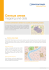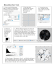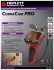# Conic-Based Algorithm for Visual Line Estimation

## Transcription

Conic-Based Algorithm for Visual Line Estimation
```Conic-Based Algorithm for Visual Line Estimation from One Image
Haiyuan Wu
Qian Chen
Faculty of Systems Engineering
Wakayama University
Wakayama City, Wakayama, 640-8510, Japan
Abstract
pupil center, or the normal vector of the supporting plane of
the iris boundaries.
Most of the gaze researches are focused on the eye detection or gaze tracking, which only give the position of the
eyes on the image plane-. Many of them assume that
the iris contours in the image are circles, which is not always true.
Mastumoto et al proposed a gaze estimation method.
They assumed that the position of the eyeball center relative to the two eye corners is known (manually adjusted),
and the iris contour is a circle. They located the eye corners
by using a binocular stereo system, and estimated the iris
center by applying Hough Transformation to the detected
iris contours. Then, they calculate the 3D position of the
eyeball center from the head pose and two eye corners. The
gaze direction is calculated from the iris center and the eyeball center.
Wang et al presented a “one-circle” algorithm for estimating the gaze direction using a monocular camera with
known focal length. They detected the elliptical iris contour and used it to calculate the normal vector of the supporting plane of the circular iris boundary. In order to resolve the ambiguity of the multi-solutions, they assumed
that the 3D distance between the eyeball center to each of
the two eye corners are equal. However, they did not show
how the 3D positions of the two eye corners were obtained.
There are two kinds of approaches to estimate the visual
line. The first one uses elliptical iris contour to estimate the
normal vector of the supporting plane of the iris boundary.
The other is to estimate the invisible eyeball center, then to
use it to calculate the visual line together with the detected
iris center.
We consider that the former is a more useful and more
reliable approach because it depends on less image features and knowledge about the eyes than the eyeball center
based algorithms. The accuracy of the conic-based algorithm depends on the precision of the elliptical iris contour
detected from an input image. In order to obtain satisfy
results, the size of the iris contour in the image should be
big enough. We consider that this requirement is an ac-
This paper describes a novel method to estimate visual line
from a single monocular image. By assuming that the visual
lines of the both eyes are parallel and the iris boundaries
are circles, we propose a “two-circle” algorithm that can
estimate the normal vector of the supporting plane of the
iris boundaries, from which the direction of the visual line
can be calculated. Most existing gaze estimation algorithms
require eye corners and some heuristic knowledge about the
structure of the eye in addition to the iris contours. In contrast to the exiting methods, our one does not use either
algorithm is that a camera with an unknown focal length
can be used without assuming the orthographical projection. This is a very useful feature because it allows one to
use a zoom lens and to change the zooming factor whenever
he or she likes. It also gives one more freedom of the camera setting because keeping the camera far from the eyes
is not necessary in our method. The extensive experiments
over simulated images and real images demonstrate the robustness and the effectiveness of our method.
1. Introduction
Gaze direction provides several functions such as giving
cues of people’s interest, attention or reference by looking
at an object or person. It also plays an important role in
many human computer interaction applications. So, the estimation of gaze direction is a very important task.
According to the anatomy, the visual line defines the
gaze direction, which is a straight line passing through the
object of fixation, the nodal point and the fovea. Because
the nodal point and the fovea are in the eyeball, they cannot be observed with a normal video camera. Therefore the
visual line cannot be estimated directly. However, since the
visual line and the line connecting the eyeball center and
the pupil center, or the visual line and the normal vector of
the supporting plane of the iris boundary form a (approximately) constant angle, the visual line can be calculated
indirectly from the 3D position of the eyeball center and the
1
ceptable because 1) High-resolution cameras are becoming popular and less expensive nowadays. For example, a
HDTV camcorder made by JVC (GR-HD1) has a resolution of 1280x720 pixel, is available at a price of about three
thousand US dollars, 2) We are developing an active camera system for observing human faces, which consists of a
camera mounted on a pan-tilt unit. The camera parameters
including focal length and the viewing direction are controlled by a computer. By using it together with a real-time
face detecting/tracking software, big face images can be obtained continuously.
In this paper, we present a “two-circle” algorithm for estimating the visual line from the iris contours detected from
one monocular image. Our “two-circle” algorithm does not
require the whole irises or their centers to be viewable, or
the radius of them. It also allows one to use a camera with
unknown focal length. Compared with the “one-circle” algorithm, our “two-circle” algorithm has two advantages: 1)
It can give an unique solution of the visual line without using eye corners, 2) It does not require a known focal length
of the camera, and it does not use the orthographical projection approximation. The second feature allows us to forget
the orthographical approximation, which does not always
stand because the eyes are not always far from the camera.
The extensive experiments over simulated images and real
images demonstrate the robustness and the effectiveness of
our method.
be described by the following equation,
Ax2e + 2Bxe ye + Cye2 + 2Dxe + 2Eye + F = 0.
(1)
A bundle of straight lines passing through the optical
center and the ellipse forms an oblique elliptical cone that
can be described by,
PT QP = 0,
where

A

Q= B
−D
f
(2)

−D
f

−E
f .
B
C
−E
f
(3)
F
f2
Q can be expressed by its normalized eigen-vectors (v 1 ,
v2 , v3 ) and eigen-values (λ 1 , λ2 , λ3 ) as following:
where
Λ
V
Q = VΛVT ,
(4)
= diag{λ1 , λ2 , λ3 }
.
= v1 v2 v3
(5)
Considering a supporting plane coordinate system that
the origin is also the optical center, but the Z-axis is defined
by the normal vector of the supporting plane of the circle to
be viewed. Then the supporting plane and the circle can be
described by the following expressions,
z = z0
,
(6)
(x − x0 )2 + (y − y0 )2 = r2
2. The “TWO-CIRCLES” Algorithm
When people look at a place that is not very near, the visual lines of the both eyes are approximately parallel, thus
the supporting planes of the both iris boundaries are also
parallel. Here, we give a “two-circles” algorithm that can
estimate the normal vector of the supporting plane(s) from
an image of two circles on the same plane or on two different but parallel planes taken by a camera with unknown
focal length. Therefore the “two-circles” algorithm can be
used to estimate the visual line when the iris contours of the
both eyes have been detected from an input image.
where (x0 , y0 , z0 ) is the center and r is the radius of the
circle. A bundle of straight lines passing through the optical center and the circle forms an oblique circular cone
described by,
(7)
Pc T Qc Pc = 0,
where


Qc = 
2.1. Elliptical cone and circular cross section
1
0
0
1
− xz00
− yz00
− xz00
− yz00
x20 +y02 −r 2
z02


.
(8)
Since the oblique circular cone Q c and the oblique elliptical cone Q are the same cone surface, there is a rotation
matrix Rc that transforms Pc to P as following,
Here, we describe the problem of estimating the direction
of a circle plane from one perspective view. M.Dhome
object of revolution. We give a rigorous description here,
from which we derive the “two-circle” algorithm.
When a circle is projected onto an image plane by perspective projection, it shows an ellipse in general case. Considering a camera coordinate system that the origin is the
optical center and the Z-axis is the optical axis, then the ellipse on the image plane z = −f (f is the focal length) can
P = Rc Pc .
(9)
Since kQc describes the same cone as of Q c for any
non-zero k, we obtain the following equation from Eq.(9),
Eq.(7), Eq(4) and Eq.(2),
(VT Rc )T Λ(VT Rc ) = kQc .
2
(10)
If we use R to indicate V T Rc as following,


r1x r2x r3x
R =  r1y r2y r3y  = VT Rc ,
r1z r2z r3z
2.2. The “TWO-CIRCLES” Algorithm
As described in the section 2.1, the normal vector of the
supporting plane and the center of the circle can be determined from one perspective image, when the focal length
is known. When the focal length is unknown, two iris contours are detected and fitted with ellipses, according to section 2.1, two oblique elliptical cones can be formed from the
each of the detected ellipses if we give a focal length. From
each of them, the normal vector of the supporting plane can
be estimated independently. If we have given a wrong focal length, the formed cones will be deformed from the real
ones, and the estimated normal vectors of the supporting
plane(s) from each of the two cones will be different from
each other. Only if we give the correct focal length, the
normal vectors estimated from each of the detected ellipses
will become parallel.
Let N1 (f ) denote the normal vector estimated from one
of the two ellipses and N 2 (f ) denote the normal vector estimated from the other. Because the supporting planes of the
two circles are parallel, N 1 (f ) and N2 (f ) are also parallel.
This constraint can be expressed by the following equation,
(11)
we have RT R = I. By substituting Eq.(11) for V T Rc in
Eq.(10), we obtain the following equations,
2
2
2
2
2
2
λ1 (r1x
− r2x
) + λ2 (r1y
− r2y
) + λ3 (r1z
− r2z
) =0
.
=0
λ1 r1x r2x + λ2 r1y r2y + λ3 r1z r2z
(12)
Without losing generality, we assume that
λ1 λ2 > 0,
λ1 λ3 < 0,
|λ1 | ≥ |λ2 |.
(13)
T
Solving Eq.(12) and R R = I, we obtain,


g cos α
S1 g sin α
S2 h
sin α
−S1 cos α
0 ,
R=
S1 S2 h cos α S2 h sin α −S1 g
(14)
where α is a free variable, S 1 and S2 are undetermined
signs, and
λ2 − λ3
λ1 − λ2
,
h=
,
(15)
g=
λ1 − λ3
λ1 − λ3
Thus Rc can be calculated as following from Eq.(11):
Rc = VR
N1 (f ) × N2 (f ) = 0.
Thus by minimizing the following expression, the normal
vector as well as the focal length f can be determined. The
undermined signs remained in Eq.(17) and Eq.(18) can also
be determined at the same time.
2
N1 (f ) × N2 (f ) → min .
(21)
(16)
Then the normal vector of the supporting plane can be
calculated as following,


 
2
S2 λλ11 −λ
0
−λ
3



N = Rc  0  = V 
(17)


0
λ2 −λ3
1
−S
1
Therefore, by taking a face image where two eyes are
viewed, the normal vector of the supporting planes of the
both circular iris boundaries can be estimated, from which
the visual line can be calculated. Here, the iris center, the
eyeball center, the iris radius and other facial features except
the iris contour are not used.
λ1 −λ3
By substituting Eq.(16) for R c in Eq.(10), k, x 0 /z0 ,
y0 /z0 and r/z0 are determined. Then the center of the circle
(denoted by C) described in the camera coordinate system
can be computed by the following expression,




λ1 −λ2
λ3
S
x0 /z0
 2 λ2 λ1 −λ3 
.
0
C = z0 Rc  y0 /z0  = z0 V 


1
−S λ1 λ2 −λ3
1 λ2
(20)
3. Experimental Results
3.1 Experiment With Simulated Images
λ1 −λ3
(18)
However, since the two undetermined signs S 1 and S2
are left, we have four possible answers for N and C. If we
define the normal vector of the supporting vector to be the
one directing to the camera, and if we know that the center
of the circle is in front of the camera, we have the following
constraints,
T
>0
N• 0 0 1
.
(19)
T
C• 0 0 1
<0
We first tested our algorithm on some simulated images to
exam the estimation error caused by the quantification error.
We used computer graphics to synthesize images of scenes
containing coplanar circles. The image resolution is 640 ×
480 [pixel].
We first set the focal length to 200 [pixel], the tilt angle θ (the angle between the optical axis and the supporting plane) to 40 [degree], the roll angle β (the rotation angle about the optical axis) to 10 [degree], and the distance
between the optical center and the supporting plane to 3.0
[meter]. We called this camera setting as “case-1” hereafter, and used it to synthesize images of circles of which
Then at least one of S 1 and S2 can be determined, thus the
number of the possible answers of N and C is at most two.
3
the radius is 1.0 [meter]. Figure 1 shows an image containing all the circles used in the experiment using the “case-1”
camera setting. We used 32 images containing two ellipses
randomly selected from the image shown in Figure 1(a).
We also used a different camera setting (“case-2”) to do
the similar experiment. This time, we set the focal length to
300 [pixel], θ = 50 [degree] and β = 30 [degree]. All other
parameters remained same as “case-1”. Figure 1(b) shows
an image containing all the circles used in the experiment of
the “case-2” camera setting. We used 17 images containing
two circles randomly placed on the supporting plane, the
ones shown in Figure 1(b).
From each image, two ellipses were detected and fitted,
which were then used to estimate the normal vector of the
supporting plane and the focal length. From the normal vector of the supporting plane, the tilt angle and the roll angle
were calculated. The estimated focal length, the tilt angle
and the roll angle were compared to the camera parameters
used to synthesize the images with CG. The experimental
results are summarized in Table 1 with suffixes 1 and 2.
We noticed that if the minor axis is greater than 30 pixels,
the estimation error will become small enough.
Table 1: Estimated camera extrinsic parameters using synthesized images
RMS error Standard deviation
f1 (pixel)
5.52
9.21
β1 (degree)
0.36
0.47
θ1 (degree)
0.57
0.97
f2 (pixel)
7.19
11.89
β2 (degree)
0.11
0.15
θ2 (degree)
0.51
0.85
(a) Original image
(b) Converted image 1-2
(c) Converted image 2-3
(d) Converted image 3-1
Figure 2: Original image and converted images.
3.2. Experiment With Real Images
(a) case-1.
We tested our method with a real image shown in Figure
2(a). It contains three circular objects: CD1 (the big CD
on the table), CD2 (the big CD on the book) and CD3
(the small CD on the table). CD1 and CD3 are coplanar, and CD2 is on another plane that is parallel to the
supporting plane of CD1 and CD3. The image size is
1600 × 1200[Pixel]. The detected and fitted ellipses are
superimposed on the original image. The parameters of the
detected ellipses are shown in Table 2, where a, b, θ and
(x0 , y0 ) is the major axis, minor axis, the angle between the
major axis and the X-axis, and the center of the ellipse, respectively. The origin of the image coordinate system is the
image center, and the Y axis directs to the upper direction.
The normal vector of the supporting plane (the table) and
the focal length of the camera can be estimated using any
two of the three circles (CD discs). The results are summarized in Table 3. Since the true answer is not available, we
used the estimation results to convert the original image to a
vertical view of the table to see if it resembles the real scene
(b) case-2.
Figure 1: images of coplanar circles synthesized by CG
4
Table 2: Estimated parameters of the ellipses
No.
CD1 CD2 CD3
a(pixel)
332
307
242
b(pixel)
180
127
162
θ(degree) 13.2 -1.1
3.5
x0 (pixel) 400 -440 -162
y0 (pixel) -115 325 -252
y
z
100cm
27
C1
Table 3: Estimated camera extrinsic parameters
image shown in Figure 2(a)
No.
θ
β
Normal Vector
(degree) (degree)
1-2
33.3
4.9
(0.07 0.83 0.55)
2-3
34.2
6.1
(0.09 0.82 0.56)
3-1
33.7
6.3
(0.09 0.83 0.55)
x
o
C2
from the
Figure 3: The experiment environment.
f
(pixel)
1998
1893
2007
Pre−processing
Binarization
Find edge
or not. The three results obtained by using different circle
pairs are shown in figure 2 (b), (c) and (d). In the converted images, each circular object shows a circle and the
book shows a rectangle. This indicates that the “two-circle”
algorithm could give correct results for real images.
(a) Original image,
(b) Detecting iris,
(c) fitted iris contours,
Image size
al : bl : θl : x0l : y0l
Visual line direction
(Pixel)
ar : br : θr : x0r : y0r
720 × 480 33: 33: -35.9: 190: 63
(-0.35 -0.07 0.94)
34: 30: 83.3: -178: 56
(d) Parameters of the fitted ellipses and the estimated
visual line direction.
3.3. Experiment With Real Face Images
We tested our method by applying it to many real face
images taken by two digital still cameras (Canon EOS
DigitalKiss) with a zoom lens (f=18-55[mm] or f=24507500[Pixel]). The image size is 3072 × 2048[Pixel]. Some
of the images used in the experiment are shown in Figure 5.
The experimental environment for taking the images No.1No.6 is shown in figure 3, where we let a user to look at a
marker far away from he/she in the frontal direction. The
image No.1-No.2 were taken by camera C1, and No.3-No.6
were taken by camera C2. The images No.7-No.9 were
pose or the fixation direction were given to users.
For each image, the eye regions are detected. Some
simple image enhancement processings are applyed to the
eye region to make the iris clear. Then the iris contours
are detected and fitted with ellipses, which are used to calculate the normal vector of the supporting plane of the iris
boundaries with the “two-circles” algorithm. The direction
of the visual line is obtained from the estimated normal vector. Figure 4 shows the procedure of visual line estimation.
The experimental results obtained from the images shown
in Figure 5 are summarized in Table 4, and estimated visual
line was showed as arrows superimposed on iris in each face
image.
Figure 4: The procedure of the visual line estimation.
4. Conclusion
This paper has presented a new method to estimate visual
line from a single monocular image. This method only
uses the iris contours and does not require the whole iris
boundaries viewable, and it does not use the information
about the iris centers. Compared with existing method, our
method does not use either the eye corners, or the heuristic knowledge about the eyeball. Another advantage of our
algorithm is that a camera with an unknown focal length
can be used without assuming the orthographical projection.
The extensive experiments over simulated images and real
images demonstrate the robustness and the effectiveness of
our method.
5
Table 4: Experimental results estimated from the images
shown in Figure 5
No.
1
2
3
4
5
6
7
8
9
Elliptical iris contours
left: al : bl : θl : x0l : y0l
right: ar : br : θr : x0r : y0r
112: 100: 84.5: 672: 243
109: 103: -79.7: -477: 195
102: 99: 38.9: 861: 102
100: 98: -83.4: -146: 84
69: 56: -73.9: 390: 291
63: 52: -79.4: -277: 313
68: 63: -89.4: 635: 89
74: 59: -85.9: 14: 78
88: 75: -76.5: 324: 492
78: 60: -81.5: -375: 469
73: 70: 86.0: 647: 97
73: 62: -83.6: -109: 54
62: 55: -63.0: -98: -175
59: 46: -68.4: -588: -160
46: 37: -5.3: 107: 79
47: 35: -26.2: -325: 66
35: 33: 62.8: 456: 53
36: 34: 22.7: 43: 77
Visual line direction
(-0.28 -0.00 0.96)
(-0.07 0.02 0.99)
No.1
No.2
No.3
No.4
No.5
No.6
(-0.57 0.13 0.81)
(-0.61 -0.01 0.79
(-0.56 0.02 0.83)
(-0.49 -0.00 0.87)
(-0.36 0.26 0.89
(-0.13 0.55 0.83)
(0.13 0.20 0.97)
References
 Q.Chen, H.Wu and T.Wada, Camera Calibration with Two
Arbitrary Coplanar Circles, ECCV, 2004. (accepted)
 J.G. Wang, E. Sung and R. Venkateswarlu, Eye Gaze Estimation from a Single Image of One Eye, ICCV’03.
No.7
 A.Criminisi, J. Shotton, A. Blake and P.H.S. Torr, Gaze Manipulation for One-to-one Teleconferencing, ICCV’03.
 D.H. Yoo, et al, Non-contact Eye Gaze Tracking System by
Mapping of Corneal Reflections, FG, 2002
 Y. Mastumoto and A. Zelinsky, presented An Algorithm for
Real-time Stereo Vision Implementation of Head Pose and
Gaze Direction Measurement, FG, pp.499-504, 2000.
 J. Zhu and J. Yang, Subpixel Eye Gaze Tracking, FG, 2002
 Y.l. Tian, K. Kanade and J.F. Cohn, Dual-state Parametric
Eye Tracking, FG, pp.110-115, 2000
 A. Schubert, Detection and Tracking of Facial Feature in
Real time Using a Synergistic Approach of Spatio-Temporal
Models and Generalized Hough-Transform Techniques, FG,
pp.116-121, 2000.
 F.D.l. Torre, Y. Yacoob and L. Davis, A Probabilistic Framework for Rigid and Non-rigid Appearance Based Tracking
and Recognition, FG, pp.491-498, 2000.
 M.Dhome, J.T.Lapreste, G.Rives and M.Richetin, Spatial
Localization of Modelled Objects of Revolution in Monocular Perspective Vision, ECCV 90, pp. 475–485, 1990.
No.8
No.9
Figure 5: Some images of real scene used the experiment.
 K.Kanatani and L.Wu, 3D Interpretation of Conics and Orthogonality, Image Understanding, Vol.58, Nov, pp. 286301, 1993.
 L.Wu and K.Kanatani, Interpretation of Conic Motion and
Its Applications, Int. Journal of Computer Vision , Vol.10,
No.1, pp. 67–84, 1993.
 A. Fitzgibbon, M.Pilu and R.B.Fisher, “Direct Least Square
Fitting of Ellipses, PAMI , Vol.21, No.5, pp. 476–480, 1999.
 X.Meng and Z.Hu, A New Easy Camera Calibration Technique Based on Circular Points, PR , Vol. 36, pp. 1155-1164,
2003.
 Yang, C., Sun, F. Hu, Z., Planar Conic Based Camera Calibration, ICPR, 2000.
 H. WU, et al, Automatic Facial Feature Points Detection
with SUSAN Operator, 12th SCIA, pp.257-263, 2001.
6
```

### Remotec Andros TM F6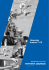### Saturday, Sept. 12, 2015 6:00 pm La Maison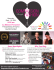### Immediate Response Information System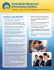### Document 6537489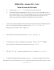### Iconix HD-RH1 Notes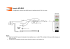### Product User Guide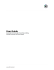### Japanese Water Iris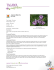### Census areas